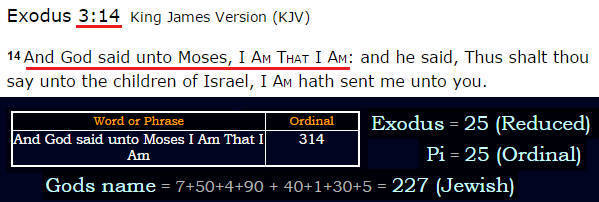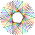CipherMar 13, 2017

Bible decodes and food for thought

I will just put up all of the bible verses I've decoded in the past and a few that I found recentlyThink about the significance of this verseAnd the Lord God said, Behold, the man is become as one of us, to know good and evil: = 8+4+5 + 7+1+4 + 6+3+9+5 + 2+3+5 + 8+8+9+5 + 7+4+1+3+6+5 + 7+1+4 + 5+8+4 + 9+8 + 7+4+6+3+5+4 + 8+8 + 3+4+4 + 3+3 + 6+8 + 7+3 + 7+4+3+4 + 2+3+3+5 + 8+4+5 + 4+5+9+6 = 322 (Reverse Reduction)

My favorite verse by far is John 21:11 - miraculous catch of fish318th prime number is 2111
21*11 = 231 (octal of 153)

7/22 = 3.18... (Second coming of Jesus Christ is mentioned 318 times in the New Testament)
22/7 = 3.14 (Jesus Christ = 151 - 227 in octal)

three hundred eighteen = 2+8+9+5+5 + 8+3+5+4+9+5+4 + 5+9+7+8+2+5+5+5 = 113 (Reduced)
second coming of Jesus Christ = 1+5+3+6+5+4 + 3+6+4+9+5+7 + 6+6 + 1+5+1+3+1 + 3+8+9+9+1+2 = 113 (Reduced)
one hundred fifty three = 6+5+5 + 8+3+5+4+9+5+4 + 6+9+6+2+7 + 2+8+9+5+5 = 113 (Reduced)
One five three = 50+40+5 + 6+9+700+5 + 100+8+80+5+5 = 1013 (Jewish)
miraculous catch of fish = 4+9+9+1+3+3+3+6+3+10 + 3+1+2+3+8 + 6+6 + 6+9+10+8 = 113 (S Exception)
fish = 6+9+90+8 = 113 (Jewish)

Fish represents Vesica Piscis, which has ratio of 265/153 or 1351/780

1³ + 5³ + 3³ = 153
Holy Bible = 19+12+15+2 + 25+18+25+15+22 = 153 (Reverse Ordinal)

153 is 17th triangular number (EL = 17, God = 17)

Another verse where 318 is mentioned in the bible has a connection to 351Genesis 14:14 is 351st verse of the bible

We see number 187 coded over and over, and there is a cipher (ALW) from Aleister Crowley that was also based on 187 but what's interesting to me is the number of chapters in Torah, from what I've seen so far Crowley was very much into Hebrew, and think about how Torah is the "Law" and how it connects to his work

There are 187 chapters in Torah
Five books of Torah = 6+9+22+5 + 2+15+15+11+19 + 15+6 + 20+15+18+1+8 = 187 (Ordinal)

English Alphabet = 25+14+11+2+23+5+4 + 1+2+26+4+1+20+25+24 = 187 (ALW)
418 was the most special number to Aleister Crowley because of his "encounter" with Aiwass, when Crowley worked out his name in gematria it had a sum of 418 which is why this number is special and also the different spelling had gematria of 93
Four One Eight = 18+7+17+12 + 7+14+25 + 25+23+11+4+24 = 187 (ALW)

187 is 11x17
187th prime number is 11171.1.